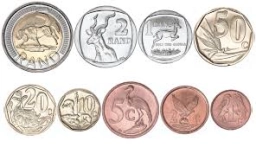# Follows: 80998

The three friends divided the money earned as follows: Tonda got 2/5, Karel 2/9, and Jirko took the rest of CZK 102. How much did each of them earn?

t =  108 Kc
k =  60 Kc

### Step-by-step explanation:Did you find an error or inaccuracy? Feel free to write us. Thank you!

Tips for related online calculators
Need help calculating sum, simplifying, or multiplying fractions? Try our fraction calculator.
Check out our ratio calculator.
Do you want to convert time units like minutes to seconds?